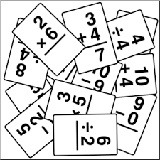Classroom Pronunciation Reductions Grammar Videos Conversation Reading Activities Listening Study Idioms Slang Acronyms Surveys Tests Alphabet Alphabet 4 Kids Vocabulary Travel America Drive AmericaFlashcards 4 Kids - IntroductionFlashcards 4 Kids - Introduction Hey this a new section of Fun Easy English. If you like these flashcards, please post a comment below. If enough of you like them then I plan to add many more. Thanks. Add Flashcards 4 Kids - to YOUR page, blog, or website. Simply cut and paste the script at the bottom of each flashcard page.
 Flashcards 4 Kids - General Alphabet - simple flashcards for learning ABCs Animals - get to know a few of your animal friends Colors - an easy way to learn basic colors Fruits - a few of the more common fruits Insects - a bunch of creepy bugs Solar System - visit our neighbors in outer space Time - Analog Full Hour Time - Digital Full Hour Time - Military Full Hour Vegetables - a group of really tasty stuff Weather - something that effects people every day
 Flashcards 4 Kids - Numbers Numbers - learn to count from 1-10 Numbers - learn to count from 11-20 Numbers - learn to count from 21-30 Numbers - learn to count from 31-40 Numbers - learn to count from 41-50 Numbers - learn to count from 51-60 Numbers - learn to count from 61-70 Numbers - learn to count from 71-80 Numbers - learn to count from 81-90 Numbers - learn to count from 91-100 Numbers - learn to count from 1-100
 Flashcards 4 Kids - Mathematics Addition - One Plus Number Addition - Two Plus Number Addition - Three Plus Number Addition - Four Plus Number Addition - Five Plus Number Addition - Six Plus Number Addition - Seven Plus Number Addition - Eight Plus Number Addition - Nine Plus Number Addition - Ten Plus Number Counting - 1-10 Division - Positive Integers Multiplication - One Times Number Multiplication - Two Times Number Multiplication - Three Times Number Multiplication - Four Times Number Multiplication - Five Times Number Multiplication - Six Times Number Multiplication - Seven Times Number Multiplication - Eight Times Number Multiplication - Nine Times Number Multiplication - Ten Times Number Subtraction - One Minus Number Subtraction - Two Minus Number Subtraction - Three Minus Number Subtraction - Four Minus Number Subtraction - Five Minus Number Subtraction - Six Minus Number Subtraction - Seven Minus Number Subtraction - Eight Minus Number Subtraction - Nine Minus Number Subtraction - Ten Minus Number Subtraction - Zero and Positive Integers